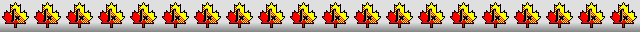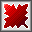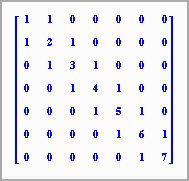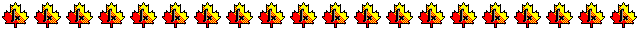Maple worksheets on linear systemsNumerical methods topics:

They are all compatible with Classic Worksheet Maple 10.Introduction - matintro.mws

• Maple data structures for vectors and matrices - new and old
• Shortcuts for entering vectors and matrices
• Operations on matrices using Maple
• Solving linear systems of equations with Maple
• Constructing random vectors and matrices
• Calculations involving matrices with floating point entries

Gaussian elimination - gauss.mws

• A procedure for performing Gaussian elimination: GaussElimination.
• Use of partial pivoting in Gaussian elimination

Gauss-Jordan elimination - gaussj.mws

• Elementary row operations and reduced echelon form
• A procedure for performing Gaussian elimination: GaussElimination.

An introduction to LU decomposition - LUintro.mws

• Finding an LU decomposition from first principles.
• Using Gauss and Gauss-Jordan elimination to find an LU decomposition
• Using LU decomposition to solve a linear system of equations

Solution of linear systems by LU decomposition - LUdecomp.mws

• LU decomposition and its application in solving linear systems.
• A procedure for constructing the LU decomposition of a non-singular matrix: LU_decomposition.
• Use of the Maple procedure LUdecomposition in the "LinearAlgebra" package.

Matrix inversion by Newton's method - newtoninv.mws

• How to divide using multiplication.
• A procedure for performing matrix inversion by Newton's method: newtoninverse.

Tridiagonal matrices - tridiag.mws

• Compact storage of tridiagonal matrices.
• A procedure which expands the compact form of a tridiagonal matrix: Tridiagonal.
• A procedure for multiplying a vector by a tridiagonal matrix in compact form: MultVector.
• A procedure for solving a linear system with a tridiagonal coefficient matrix: TridiagSolve.

Construction of Matrices with random entries - randmat.mws

• Procedures for generating random numbers: randuniform, randnormal.
• Using Maple procedures in "stats[random,...]" to generate random numbers.
• A procedure for constructing random data distributions: distributiondata.
• Graphing distributions.
• Constructing random vectors and matrices.

Procedures for linear systems - linsys.zipTop of page

Main index